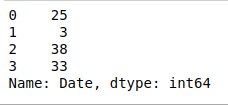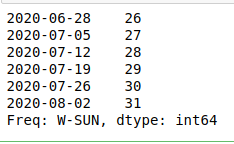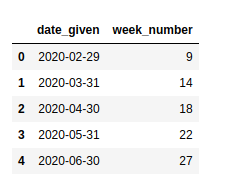# Extract week number from date in Pandas-Python

• Last Updated : 10 Jul, 2020

Many times, when working with some data containing dates we may need to extract the week number from a particular date. In Python, it can be easily done with the help of pandas.

Example 1:

 `# importing pandas as pd ` `import` `pandas as pd  ` ` `  `# creating a dictionary containing a date ` `dict` `=` `{``'Date'``:[``"2015-06-17"``]} ` ` `  `# converting the dictionary to a dataframe ` `df ``=` `pd.DataFrame.from_dict(``dict``) ` ` `  `# converting the date to the required format ` `df[``'Date'``] ``=` `pd.to_datetime(df[``'Date'``], errors ``=``'coerce'``) ` `df.astype(``'int64'``).dtypes ` ` `  `# extracting the week from the date ` `weekNumber ``=` `df[``'Date'``].dt.week ` ` `  `print``(weekNumber) `

Output:

```0    25
Name: Date, dtype: int64```

Example 2: We can also do the same for multiple dates by adding more dates in the ‘Date’ object.

 `# importing pandas as pd ` `import` `pandas as pd  ` ` `  `# creating a dictionary containing a date ` `dict` `=` `{``'Date'``:[``"2020-06-17"``, ``"2020-01-14"``,  ` `                ``"2020-09-20"``, ``"2020-08-15"``]} ` ` `  `# converting the dictionary to a  ` `# dataframe ` `df ``=` `pd.DataFrame.from_dict(``dict``) ` ` `  `# converting the date to the required  ` `# format ` `df[``'Date'``] ``=` `pd.to_datetime(df[``'Date'``], ` `                            ``errors ``=``'coerce'``) ` `df.astype(``'int64'``).dtypes ` ` `  `# extracting the week from the date ` `weekNumber ``=` `df[``'Date'``].dt.week ` ` `  `print``(weekNumber) `

Output:Example 3: Extracting week number from dates for multiple dates using date_range() and to_series().

• pandas.data_range(): It generates all the dates from the start to end date

Syntax:

pandas.date_range(start, end, periods, freq, tz, normalize, name, closed)

• pandas.to_series(): It creates a Series with both index and values equal to the index keys.

Syntax:

`Index.to_series(self, index, name)`

 `# importing pandas as pd ` `import` `pandas as pd  ` ` `  `# generating all dates in given range ` `# with increment by days ` `allDates ``=` `pd.date_range(``'2020-06-27'``, ``'2020-08-03'``, freq ``=``'W'``) ` ` `  `# converting dates to series ` `series ``=` `allDates.to_series() ` ` `  `series.dt.week `

Output:Example 4: In this example, we’ll be using pandas.Series() to generate dates and use a different way to convert the series to the dataframe.

pandas.Series(): Used to create a one-dimensional ndarray with axis labels.
Syntax:

`pandas.Series(data, index, dtype, name, copy, fastpath)`

 `# importing pandas as pd ` `import` `pandas as pd  ` ` `  `# generating the series ` `dates ``=` `pd.Series(pd.date_range(``'2020-2-10'``, ` `                                ``periods ``=` `5``, ` `                                ``freq ``=``'M'``)) ` ` `  `# converting to dataframe ` `df ``=` `pd.DataFrame({``'date_given'``: dates}) ` ` `  `# extracting the week number ` `df[``'week_number'``] ``=` `df[``'date_given'``].dt.week ` ` `  `df `

Output:My Personal Notes arrow_drop_up
Recommended Articles
Page :